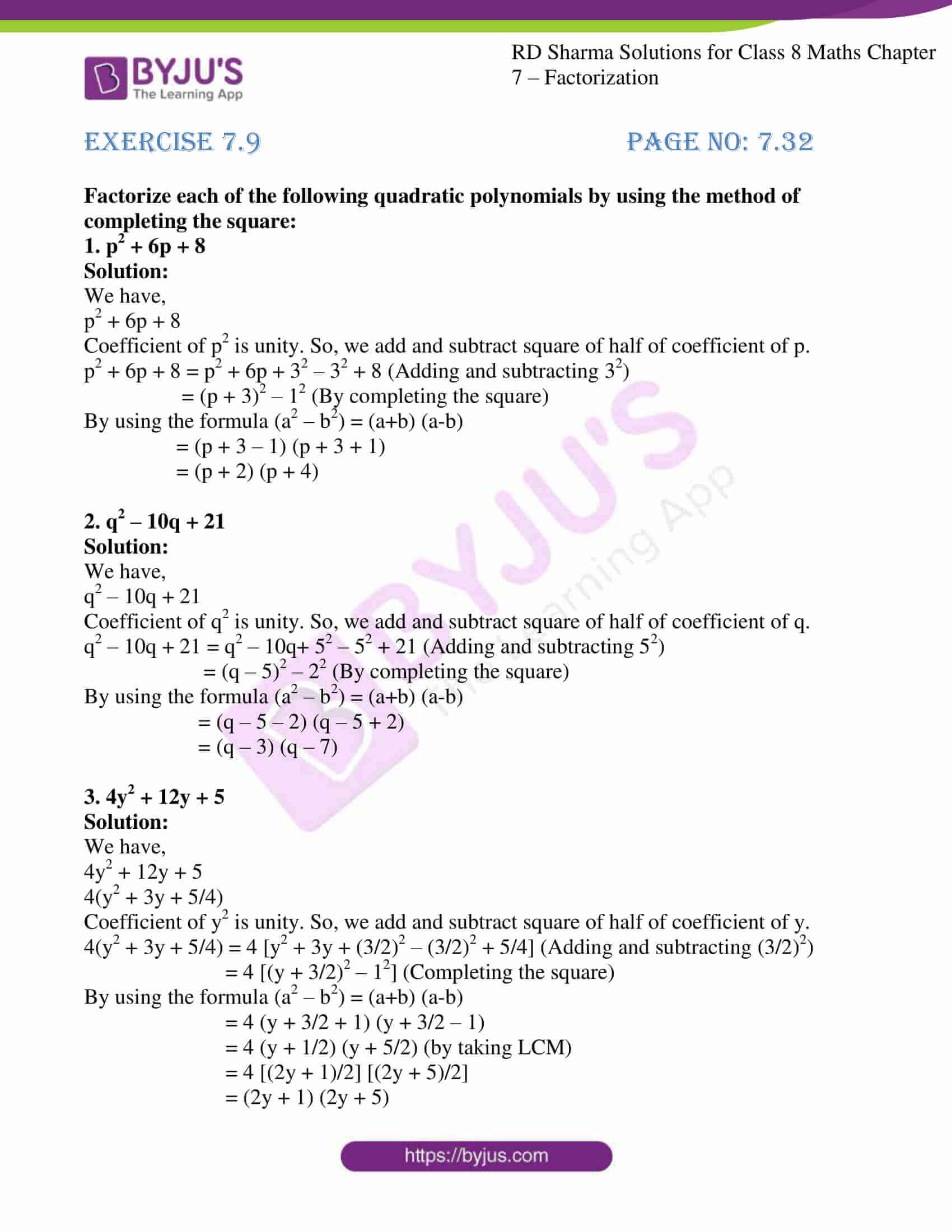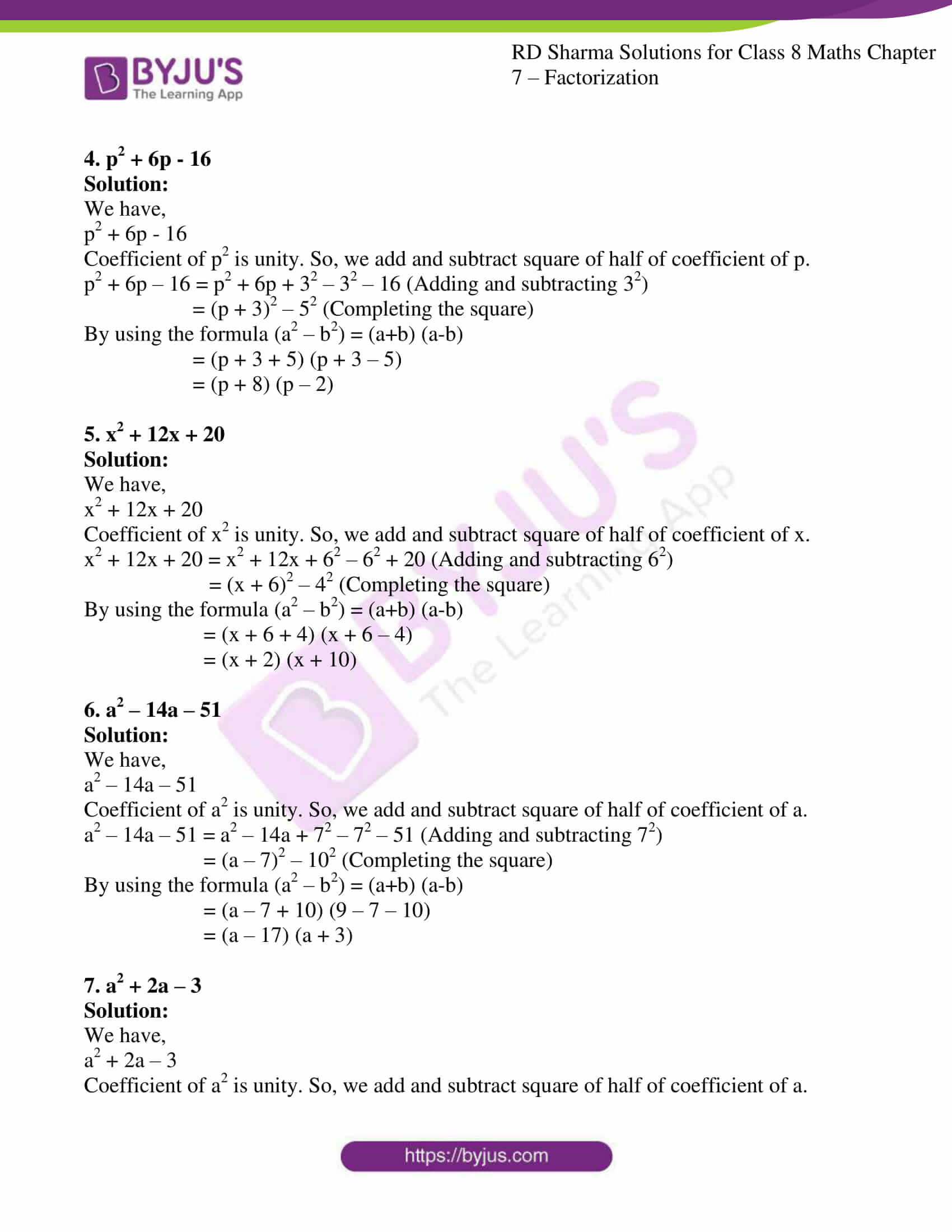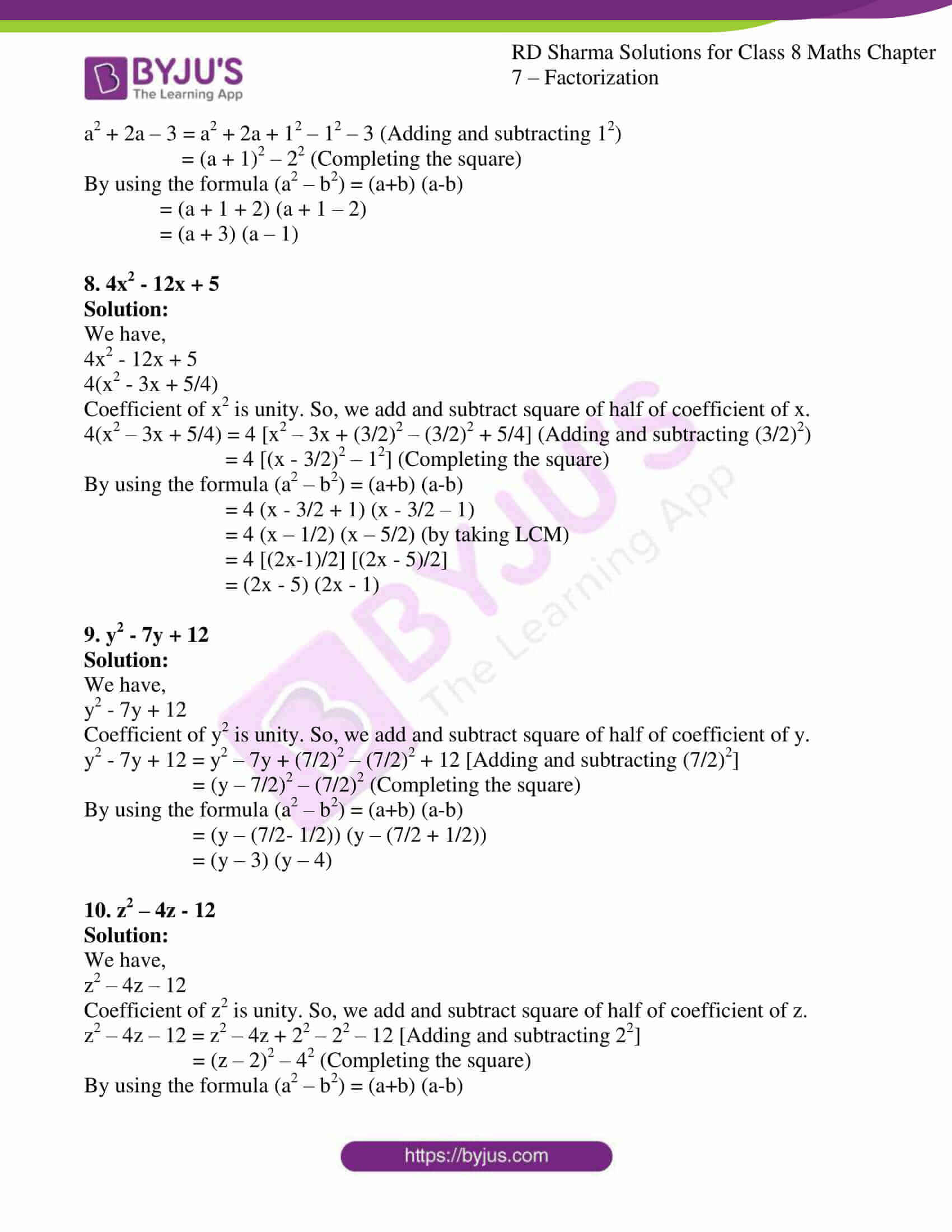# RD Sharma Solutions for Class 8 Maths Chapter - 7 Factorization Exercise 7.9

To make learning easy for students RD Sharma Solutions for Class 8 Maths Chapter 7 Factorization Exercise 7.9 are provided here. In this Exercise 7.9 of RD Sharma, we shall discuss the problems based on the factorization of quadratic polynomials by using the method of completing the perfect square. Our experts have formulated textbook solutions in a clear manner to help students prepare for their board exams and come out with flying colours in their examinations. Students can download free pdf of RD Sharma Solutions from the links provided below.

## Download the pdf of RD Sharma Solutions For Class 8 Maths Exercise 7.9 Chapter 7 Factorization### Access Answers to RD Sharma Solutions for Class 8 Maths Exercise 7.9 Chapter 7 Factorization

#### EXERCISE 7.9 PAGE NO: 7.32

Factorize each of the following quadratic polynomials by using the method of completing the square:

1. p2 + 6p + 8

Solution:

We have,

p2 + 6p + 8

Coefficient of p2 is unity. So, we add and subtract square of half of coefficient of p.

p2 + 6p + 8 = p2 + 6p + 32 – 32 + 8 (Adding and subtracting 32)

= (p + 3)2 – 12 (By completing the square)

By using the formula (a2 – b2) = (a+b) (a-b)

= (p + 3 – 1) (p + 3 + 1)

= (p + 2) (p + 4)

2. q2 – 10q + 21

Solution:

We have,

q2 – 10q + 21

Coefficient of q2 is unity. So, we add and subtract square of half of coefficient of q.

q2 – 10q + 21 = q2 – 10q+ 52 – 52 + 21 (Adding and subtracting 52)

= (q – 5)2 – 22 (By completing the square)

By using the formula (a2 – b2) = (a+b) (a-b)

= (q – 5 – 2) (q – 5 + 2)

= (q – 3) (q – 7)

3. 4y2 + 12y + 5

Solution:

We have,

4y2 + 12y + 5

4(y2 + 3y + 5/4)

Coefficient of y2 is unity. So, we add and subtract square of half of coefficient of y.

4(y2 + 3y + 5/4) = 4 [y2 + 3y + (3/2)2 – (3/2)2 + 5/4] (Adding and subtracting (3/2)2)

= 4 [(y + 3/2)2 – 12] (Completing the square)

By using the formula (a2 – b2) = (a+b) (a-b)

= 4 (y + 3/2 + 1) (y + 3/2 – 1)

= 4 (y + 1/2) (y + 5/2) (by taking LCM)

= 4 [(2y + 1)/2] [(2y + 5)/2]

= (2y + 1) (2y + 5)

4. p2 + 6p – 16

Solution:

We have,

p2 + 6p – 16

Coefficient of p2 is unity. So, we add and subtract square of half of coefficient of p.

p2 + 6p – 16 = p2 + 6p + 32 – 32 – 16 (Adding and subtracting 32)

= (p + 3)2 – 52 (Completing the square)

By using the formula (a2 – b2) = (a+b) (a-b)

= (p + 3 + 5) (p + 3 – 5)

= (p + 8) (p – 2)

5. x2 + 12x + 20

Solution:

We have,

x2 + 12x + 20

Coefficient of x2 is unity. So, we add and subtract square of half of coefficient of x.

x2 + 12x + 20 = x2 + 12x + 62 – 62 + 20 (Adding and subtracting 62)

= (x + 6)2 – 42 (Completing the square)

By using the formula (a2 – b2) = (a+b) (a-b)

= (x + 6 + 4) (x + 6 – 4)

= (x + 2) (x + 10)

6. a2 – 14a – 51

Solution:

We have,

a2 – 14a – 51

Coefficient of a2 is unity. So, we add and subtract square of half of coefficient of a.

a2 – 14a – 51 = a2 – 14a + 72 – 72 – 51 (Adding and subtracting 72)

= (a – 7)2 – 102 (Completing the square)

By using the formula (a2 – b2) = (a+b) (a-b)

= (a – 7 + 10) (9 – 7 – 10)

= (a – 17) (a + 3)

7. a2 + 2a – 3

Solution:

We have,

a2 + 2a – 3

Coefficient of a2 is unity. So, we add and subtract square of half of coefficient of a.

a2 + 2a – 3 = a2 + 2a + 12 – 12 – 3 (Adding and subtracting 12)

= (a + 1)2 – 22 (Completing the square)

By using the formula (a2 – b2) = (a+b) (a-b)

= (a + 1 + 2) (a + 1 – 2)

= (a + 3) (a – 1)

8. 4x2 – 12x + 5

Solution:

We have,

4x2 – 12x + 5

4(x2 – 3x + 5/4)

Coefficient of x2 is unity. So, we add and subtract square of half of coefficient of x.

4(x2 – 3x + 5/4) = 4 [x2 – 3x + (3/2)2 – (3/2)2 + 5/4] (Adding and subtracting (3/2)2)

= 4 [(x – 3/2)2 – 12] (Completing the square)

By using the formula (a2 – b2) = (a+b) (a-b)

= 4 (x – 3/2 + 1) (x – 3/2 – 1)

= 4 (x – 1/2) (x – 5/2) (by taking LCM)

= 4 [(2x-1)/2] [(2x – 5)/2]

= (2x – 5) (2x – 1)

9. y2 – 7y + 12

Solution:

We have,

y2 – 7y + 12

Coefficient of y2 is unity. So, we add and subtract square of half of coefficient of y.

y2 – 7y + 12 = y2 – 7y + (7/2)2 – (7/2)2 + 12 [Adding and subtracting (7/2)2]

= (y – 7/2)2 – (7/2)2 (Completing the square)

By using the formula (a2 – b2) = (a+b) (a-b)

= (y – (7/2- 1/2)) (y – (7/2 + 1/2))

= (y – 3) (y – 4)

10. z2 – 4z – 12

Solution:

We have,

z2 – 4z – 12

Coefficient of z2 is unity. So, we add and subtract square of half of coefficient of z.

z2 – 4z – 12 = z2 – 4z + 22 – 22 – 12 [Adding and subtracting 22]

= (z – 2)2 – 42 (Completing the square)

By using the formula (a2 – b2) = (a+b) (a-b)

= (z – 2 + 4) (z – 2 – 4)

= (z – 6) (z + 2)